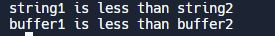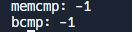×

Search anything:

# memcmp() function in C++

#### C++ C Programmingmemcmp() is a C++ function that compares a specified number of characters in two pointer objects.

It Compares the first number of bytes in the memory block pointed to by "pointer1" with the first number of bytes pointed to by "pointer2", returning zero if both match or a non-zero value if they do not.

This built-in function compares the first byte of buf1 and buf2. The memcmp function compares the first n bytes of s1 and s2 as unsigned character arrays. If all n bytes are equal, the return value is 0. Otherwise, if s1 is greater than s2, the return value is positive; If s1 is less than s2, the return value is negative.

Although unlike the strcmp() function in C++, this function does not stop comparing after encountering a null character.

The syntax of memcmp looks like this:

``````int memcmp(const void *ptr1, const void *ptr2, size_t num);
``````

This function compares the first num bytes of the memory blocks pointed to by ptr1 and ptr2, and num is the number of bytes to compare.

The parameters of memcmp() function are:

1. ptr1, ptr2 - It points to the memory buffers which are being compared.

2. num - The total no of data being examined.

The Return value of memcmp() functions can return three kinds of output, they are -

1. Positive value - If the value of the first differing bytes present in the LHS pointer being compared is greater than the value of the first differing bytes present in the RHS pointer being compared, the memcmp function() will return a positive value.

2. Negative value - If the value of the first differing bytes present in the LHS pointer being compared is lesser than the value of the first differing bytes present in the RHS pointer being compared, the memcmp function() will return a negative value.

3. Null value - If the value of the first differing bytes of the LHS pointer being compared is equal to the value of the first differing bytes present in the RHS pointer being compared, the memcmp function() will return a null or 0 value.

# The Difference between strncmp and memcmp is :

The strncmp() and memcmp() functions are both used to compare two blocks of memory in C++. However, there are several key differences between these two functions:

1. The strncmp() function is used to compare null-terminated strings, while the memcmp() function can be used to compare any two blocks of memory.

2. The strncmp() function compares the characters in the strings one by one, starting from the first character, until it reaches the end of either string or the specified maximum number of characters. The memcmp() function, on the other hand, compares the entire memory blocks byte-by-byte, unless a non-matching byte is found.

3. The strncmp() function returns a positive, negative, or zero value to indicate the result of the comparison. The memcmp() function, on the other hand, returns an integer value that indicates the result of the comparison.

Overall, the strncmp() function is more suitable for comparing strings, while the memcmp() function is more flexible and can be used to compare any two blocks of memory.

The differences between memcmp() and strcmp() can be highlighted in the following code :

``````
#include <iostream>
#include <string.h> // for memcmp() and strcmp()

int main() {
// compare two strings using strcmp()
char string1[] = "Hello";
char string2[] = "World";
int result = strcmp(string1, string2);
if (result == 0) {
std::cout << "string1 is equal to string2" << std::endl;
} else if (result < 0) {
std::cout << "string1 is less than string2" << std::endl;
} else {
std::cout << "string1 is greater than string2" << std::endl;
}

// compare two memory blocks using memcmp()
char buffer1[] = {'H', 'e', 'l', 'l', 'o'};
char buffer2[] = {'W', 'o', 'r', 'l', 'd'};
result = memcmp(buffer1, buffer2, 5);
if (result == 0) {
std::cout << "buffer1 is equal to buffer2" << std::endl;
} else if (result < 0) {
std::cout << "buffer1 is less than buffer2" << std::endl;
} else {
std::cout << "buffer1 is greater than buffer2" << std::endl;
}

return 0;
}

``````

output :The strcmp() function is used to compare two strings, whereas the memcmp() function is used to compare two memory blocks.
In both cases, the function returns 0 if the two blocks or strings are equal, a negative value if the first block or string is less than the second, and a positive value if the first is greater than the second.

# Comparison between memcmp() and bcmp() :

1. memcmp() and bcmp() are both C standard library functions that are used to compare two blocks of memory. They both take pointers to the two blocks of memory that are to be compared, as well as the size of the blocks in bytes.

2. The main difference between these two functions is that memcmp() returns an integer value indicating the result of the comparison, while bcmp() returns a non-zero value if the two blocks of memory are different, and 0 if they are the same.

This means that bcmp() is a bit simpler to use, as you only need to check the return value to determine whether the two blocks of memory are the same or not, whereas with memcmp() you need to check the specific value of the return code to determine the result of the comparison.

3. In general, memcmp() is considered to be more flexible and powerful than bcmp(), as it allows you to compare blocks of memory of different sizes and types, and it provides more detailed information about the result of the comparison. However, bcmp() may be easier to use in some cases, especially when you only need to determine whether two blocks of memory are the same or not.

Example of the difference between memcmp() and bcmp() in terms of code :

``````#include <iostream>
#include <cstring>

int main() {
// Define two arrays of characters to compare
char a1 = "hello";
char a2 = "world";

// Use memcmp to compare the two arrays
int result1 = memcmp(a1, a2, 5);

// Use bcmp to compare the two arrays
int result2 = bcmp(a1, a2, 5);

// Print the results of the comparisons
std::cout << "memcmp: " << result1 << std::endl;
std::cout << "bcmp: " << result2 << std::endl;

return 0;
}
``````

Output :Both memcmp() and bcmp() are used to compare two arrays of characters. However, memcmp() is a standard C function, while bcmp() is a non-standard function that is specific to certain operating systems (such as BSD-based systems).

# Optimization of memcmp() -

There are several ways to optimize the memcmp() function in C++. Some possible optimization techniques are:

1. Use the memcmp() function only for comparing small memory blocks, as it can be slower than other methods for large blocks.

2. Try to use the memcmp() function to compare entire memory blocks, as it will stop comparing as soon as it finds a non-matching byte.

3. The memcmp() function should be used to compare the memory blocks byte-by-byte, as it does not take into account the data types or structure of the memory blocks.

4. memcmp() should be used to compare large memory blocks, its performance by using a faster algorithm, such as the Boyer-Moore string search algorithm.

# Complexity of memcmp() -

1. The complexity of the memcmp() function in C++ depends on the size of the arrays that are being compared.

2. In the general case, where the arrays have a size of n, the complexity of memcmp() is O(n), which means that the running time of the function grows linearly with the size of the arrays.

If you double the number of bytes to compare, the time taken by the memcmp() function will also double.

3. memcmp() can be slower than other methods for comparing large memory blocks, as it does not take advantage of any algorithmic optimization techniques.

Hence, it is best to use memcmp() only for comparing small memory blocks, or when you need to compare the entire memory blocks byte-by-byte.

With the help of this article at OpenGenus, you will have an idea on what the memcmp() function is, what it does, and how to implement it in C++.